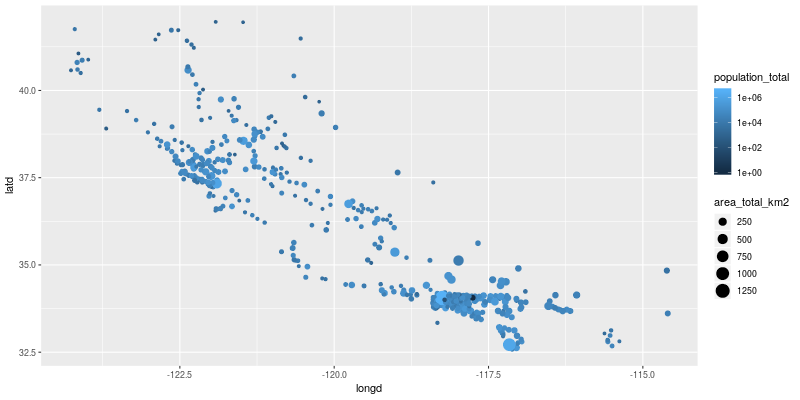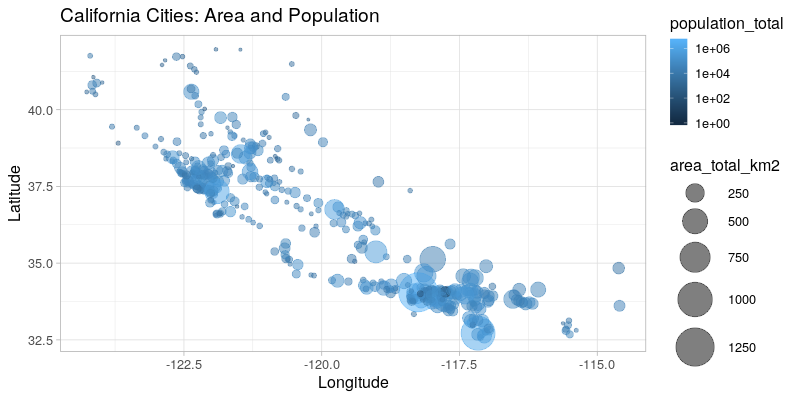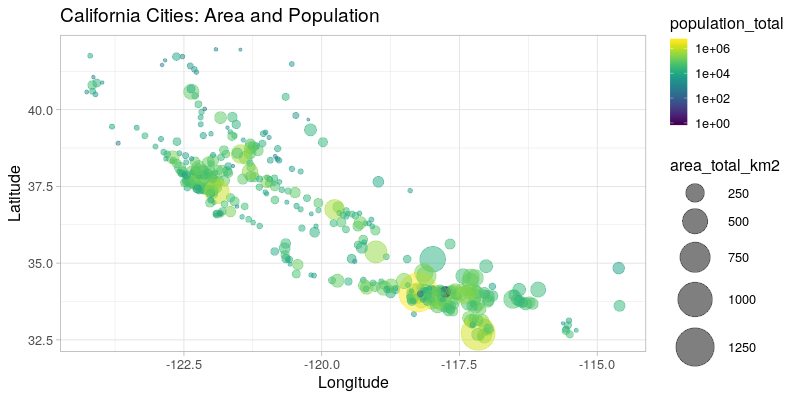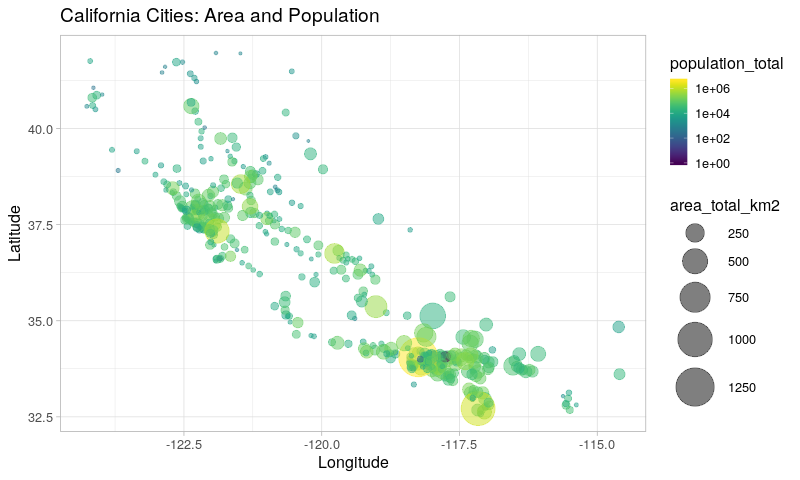In :
from functools import partial
from rpy2.ipython import html
html.html_rdataframe=partial(html.html_rdataframe, table_class="docutils")

/home/laurent/Desktop/software/python/py36_env/lib/python3.6/site-packages/rpy2-3.2.7-py3.6-linux-x86_64.egg/rpy2/robjects/pandas2ri.py:14: FutureWarning: pandas.core.index is deprecated and will be removed in a future version.  The public classes are available in the top-level namespace.
from pandas.core.index import Index as PandasIndex
/home/laurent/Desktop/software/python/py36_env/lib/python3.6/site-packages/rpy2-3.2.7-py3.6-linux-x86_64.egg/rpy2/robjects/pandas2ri.py:34: UserWarning: pandas >= 1.0 is not supported.
warnings.warn('pandas >= 1.0 is not supported.')


# Data Import¶

We choose to use an external dataset to demonstrate how R's own data import features can be used.

In :
from rpy2.robjects.packages import importr
utils = importr('utils')

'master/notebooks/data/california_cities.csv')


The objects returned by R's own read.csv() function (note that the R function in the R package utils is called read.csv() while the Python function is called read_csv() - rpy2 converts R symbols with dots to underscores for Python).

rpy2 provides customization to display R objects such as data frames in HTML in a notebook. That customization is enabled as follows:

In :
import rpy2.ipython.html
rpy2.ipython.html.init_printing()

In :
dataf

Out:
DataFrame with 482 rows and 14 columns:
X city latd longd ... area_water_km2 area_water_percent
0 1 0 Adelanto 34.57611111111112 -117.43277777777779 ... 0.046 0.03
1 2 1 AgouraHills 34.15333333333333 -118.76166666666667 ... 0.076 0.37
2 3 2 Alameda 37.75611111111111 -122.27444444444444 ... 31.983 53.79
3 4 3 Albany 37.886944444444445 -122.29777777777778 ... 9.524 67.28
4 5 4 Alhambra 34.081944444444446 -118.135 ... 0.003 0.01
5 6 5 AlisoViejo 33.575 -117.72555555555556 ... 0.0 0.0
6 7 6 Alturas 41.48722222222222 -120.5425 ... 0.036000000000000004 0.57
7 8 7 AmadorCity 38.419444444444444 -120.82416666666666 ... 0.0 0.0
... ... ... ... ... ... ... ... ...
480 481 480 Yucaipa 34.030277777777776 -117.04861111111111 ... 0.013000000000000001 0.02
481 482 481 YuccaValley 34.13333333333333 -116.41666666666667 ... 0.0 0.0
In :
dataf.colnames

Out:
StrVector with 14 elements:
 X city latd longd elevation_m elevation_ft population_total area_total_sq_mi ... area_water_km2 area_water_percent
In :
stats = importr('stats')
base = importr('base')
stats.lm('elevation_m ~ latd + longd', data=dataf)

Out:
ListVector with 13 elements:
 0 coefficients (Intercep... 1 residuals ... 2 effects ... 3 rank  3 4 fitted.values ... 5 assign  0 1 2 6 qr $qr ... 7 df.residual  431 ... ... ... 11 terms elevation... 12 model ... # Graphics¶ R has arguably some the best static visualizations, often looking more polished than other visualization systems and this without the need to spend much effort on them. ## Using ggplot2¶ Among R visulization pacakges, ggplot2 has emerged as something Python users wished so much they had that various projects to try port it to Python are regularly started. However, the best way to have ggplot2 might be to use ggplot2 from Python. In : import rpy2.robjects.lib.ggplot2 as gp  Calling ggplot2 looks pretty much like it would in R, which allows one to use the all available documentation and examples available for the R package. Remember that this is not a reimplementation of ggplot2 with inevitable differences and delay for having the latest changes: the R package itself is generating the figures. In : p = (gp.ggplot(dataf) + gp.aes_string(x='longd', y='latd', color='population_total', size='area_total_km2') + gp.geom_point() + gp.scale_color_continuous(trans='log10'))  Plotting the resulting R/ggplot2 object into the output cell of a notebook, is just function call away. In : from rpy2.ipython.ggplot import image_png image_png(p)  Out:All features from ggplot2 should be present. A more complex example to get the figure we want is: In : from rpy2.robjects.vectors import IntVector p = (gp.ggplot(dataf) + gp.aes_string(x='longd', y='latd', color='population_total', size='area_total_km2') + gp.geom_point(alpha=0.5) + # Axis definitions. gp.scale_x_continuous('Longitude') + gp.scale_y_continuous('Latitude') + # Custom size range. gp.scale_size(range=IntVector([1, 18])) + # Transform for pop -> color mapping gp.scale_color_continuous(trans='log10') + # Title. gp.ggtitle('California Cities: Area and Population') + # Plot theme and text size. gp.theme_light(base_size=16)) image_png(p)  Out:## Using ggplot2 extensions¶ There existing additional R packages extending ggplot2, and while it would be impossible for the rpy2 to provide wrapper for all of them the wrapper for ggplot2 is based on class hierarchies that should make the use of such extensions really easy. For example, to use the viridis color scale, we just need to import the corresponding R package, and write 3 lines of Python to extend rpy2's ggplot2 wrapper with a new color scale. A clas diagram with the classes in the rpy2 wrapper for ggplot2 is available in the rpy2 documentation. In : viridis = importr('viridis') class ScaleColorViridis(gp.ScaleColour): _constructor = viridis.scale_color_viridis scale_color_viridis = ScaleColorViridis.new  That new color scale can then be used as any other scale already present in ggplot2: In : p = (gp.ggplot(dataf) + gp.aes_string(x='longd', y='latd', color='population_total', size='area_total_km2') + gp.geom_point(alpha=0.5) + gp.scale_x_continuous('Longitude') + gp.scale_y_continuous('Latitude') + gp.scale_size(range=IntVector([1, 18])) + scale_color_viridis(trans='log10') + gp.ggtitle('California Cities: Area and Population') + gp.theme_light(base_size=16)) image_png(p)  Out:So far we have shown that using ggplot2 can be done from Python as if it was just an other Python library for visualization, but R can also be used in cells. First the so-called "R magic" extension should be loaded. In : %load_ext rpy2.ipython  From now on, code cells starting with %%R will see their content evaluated as R code. If the R code is generating figures, they will be displayed along with the rest of the output. In : %%R R.version.string   "R version 3.6.1 Patched (2019-09-06 r77160)"  In : %%R -i dataf require(dplyr) glimpse(dataf)  R[write to console]: Loading required package: dplyr R[write to console]: Attaching package: ‘dplyr’ R[write to console]: The following objects are masked from ‘package:stats’: filter, lag R[write to console]: The following objects are masked from ‘package:base’: intersect, setdiff, setequal, union  Observations: 482 Variables: 14$ X                  <int> 0, 1, 2, 3, 4, 5, 6, 7, 8, 9, 10, 11, 12, 13, 14, …
$city <fct> Adelanto, AgouraHills, Alameda, Albany, Alhambra, …$ latd               <dbl> 34.57611, 34.15333, 37.75611, 37.88694, 34.08194, …
$longd <dbl> -117.4328, -118.7617, -122.2744, -122.2978, -118.1…$ elevation_m        <dbl> 875, 281, NA, NA, 150, 127, 1332, 280, 14, 48, 132…
$elevation_ft <dbl> 2871, 922, 33, 43, 492, 417, 4370, 919, 46, 157, 4…$ population_total   <int> 31765, 20330, 75467, 18969, 83089, 47823, 2827, 18…
$area_total_sq_mi <dbl> 56.027, 7.822, 22.960, 5.465, 7.632, 7.472, 2.449,…$ area_land_sq_mi    <dbl> 56.009, 7.793, 10.611, 1.788, 7.631, 7.472, 2.435,…
$area_water_sq_mi <dbl> 0.018, 0.029, 12.349, 3.677, 0.001, 0.000, 0.014, …$ area_total_km2     <dbl> 145.107, 20.260, 59.465, 14.155, 19.766, 19.352, 6…
$area_land_km2 <dbl> 145.062, 20.184, 27.482, 4.632, 19.763, 19.352, 6.…$ area_water_km2     <dbl> 0.046, 0.076, 31.983, 9.524, 0.003, 0.000, 0.036, …
\$ area_water_percent <dbl> 0.03, 0.37, 53.79, 67.28, 0.01, 0.00, 0.57, 0.00, …


The data frame called dataf in our Python notebook was already bound to the name dataf in the R main namespace (GlobalEnv in the R lingo) in our previous cell. We can just use it in subsequent cells.

In :
%%R -w 800 --type=cairo

cat("Running an R code cell.\n")

p <- ggplot(dataf) +
aes_string(x = 'longd',
y = 'latd',
color = 'population_total',
size = 'area_total_km2') +
geom_point(alpha = 0.5) +
scale_x_continuous('Longitude') +
scale_y_continuous('Latitude') +
scale_size(range = c(1, 18)) +
scale_color_viridis(trans='log10') +
ggtitle('California Cities: Area and Population') +
theme_light(base_size=16)
print(p)

Running an R code cell.